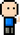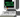# Josh Meets Computer# Clustering Measurements

April 13, 2018

Clustering measurements provide a good way to predict where future edges will be created in a graph. One strong indication of future connections is the existence of Triadic closures around nodes.

Existing connections indicate a high probability of where a new connection will be added — two nodes that share a connection have a high probability of becoming connected themselves/

## Local Clustering Coefficient

This measurement can be computed on a node by the number of pairs of connections divided by the number of pairs of connections from the nodes connections.

``````import networkx as nx

G = nx.karate_club_graph()

def local_clustering(G, n):
neighbors = G.degree(n)
pairs_neighbors = neighbors * (neighbors - 1) / 2 if neighbors > 1 else 1
friendly_neighbors = 0

for i in G.neighbors(n):
for j in G.neighbors(i):
if j in G.neighbors(n):
friendly_neighbors += 1
friendly_neighbors /= 2

coeff = friendly_neighbors / pairs_neighbors
return coeff

local_clustering(G, 31) == nx.clustering(G, 31)``````

## Global Clustering Coefficient

Can be described by taking the average of all the local clustering coefficients from each node, or by transivity — number of triangles divided by the number of open triads.

``````def ave_clustering(G):
coeff = 0
num_nodes = nx.number_of_nodes(G)

for n in G.nodes():
coeff += local_clustering(G, n)
coeff /= num_nodes

return coeff

ave_clustering(G) == nx.average_clustering(G)``````